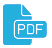# Author Details

## Malykh, Mikhail D.

 Issue Section Title File Vol 27, No 3 (2019) Computational modeling and simulation On the properties of numerical solutions of dynamical systems obtained using the midpoint method(Eng) Vol 28, No 1 (2020) Mathematical Modeling Numerical determination of the singularity order of a system of differential equations(Eng) Vol 28, No 1 (2020) Mathematical Modeling Calculation of the normal modes of closed waveguides(Eng) Vol 28, No 2 (2020) Mathematical models in Physics Kinematic support modeling in Sage(Eng) Vol 28, No 4 (2020) Articles On the realization of explicit Runge-Kutta schemes preserving quadratic invariants of dynamical systems(Eng) Vol 29, No 1 (2021) Articles Normal modes of a waveguide as eigenvectors of a self-adjoint operator pencil(Eng) Vol 29, No 1 (2021) Articles On conjugate difference schemes: the midpoint scheme and the trapezoidal scheme(Eng) Vol 29, No 3 (2021) Articles Richardson-Kalitkin method in abstract description(Eng) Vol 29, No 4 (2021) Articles On involutive division on monoids(Eng) Vol 30, No 1 (2022) Articles On the many-body problem with short-range interaction(Eng) Vol 30, No 2 (2022) Articles Complex eigenvalues in Kuryshkin-Wodkiewicz quantum mechanics(Eng) Vol 30, No 4 (2022) Articles On a dispersion curve of a waveguide filled with inhomogeneous substance(Eng)